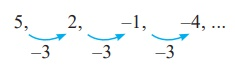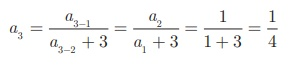Home | | Maths 10th Std | Sequences

# Sequences

A real valued sequence is a function defined on the set of natural numbers and taking real values

Sequences

Consider the following picturesThere is some pattern or arrangement in these pictures. In the first picture, the first row contains one apple, the second row contains two apples and in the third row there are three apples etc... The number of apples in each of the rows are 1, 2, 3, ...

In the second picture each step have 0.5 feet height. The total height of the steps from the base are 0.5 feet,1 feet, 1.5 feet,... In the third picture one square, 3 squares, 5 squares, ....

These numbers belong to category called “Sequences”.

### Definition

A real valued sequence is a function defined on the set of natural numbers and taking real values

Each element in the sequence is called a term of the sequence. The element in the first position is called the first term of the sequence. The element in the second position  is called second term of the sequence and so on’

If the nth  term is denoted by an, then a1  is the first term, a2  is the second term, and so on.

A sequence can be written as a1, a2, a3, …… an, …

### Illustration

1. 1,3,5,7,... is a sequence with general term an = 2n − 1 . When we put n = 1, 2, 3,..., we get a1 =1, a2 = 3, a3 = 5, a4 = 7,...

2. 1/2 , 1/3 , 1/4 , 1/5 ,... is a sequence with general term 1/ [n + 1] . When we put n = 1,2,3,.... we get

a1  = 1/2 ,  a2  = 1/3 , a3  = 1/4 , a4  = 1/5 ,...

If the number of elements in a sequence is finite then it is called a Finite sequence.  If the number of elements in a sequence is infinite then it is called an Infinite sequence

## Sequence as a Function

A sequence can be considered as a function defined on the set of natural numbers N. In particular, a sequence is a function f : N R , where R is the set of all real numbers.If the sequence is of the form a1,a2,a3,...  then we can associate the function to the sequence a1,a2,a3,... by f (k) = ak , k = 1,2,3,...

Example 2.19

Find the next three terms of the sequences

(i) 1/16 , 1/6 ,  1/14 , . . . .  (ii) 5, 2,- 1, -4,. . . . (iii) 1, 0.1, 0.01,. . .

Solution

(i)In the above sequence the numerators are same and the denominator is increased by 4.

So the next three terms are(ii)Here each term is decreased by 3. So the next three terms are -7,  -10,  -13 .

(iii)Here each term is divided by 10. Hence, the next three terms arenote

Though all the sequences are functions, not all the functions are sequences.

Example 2.20

Find the general term for the following sequences

(i) 3, 6, 9,...  (ii) 1/2 , 2/3 , 3/4 ,... (iii) 5, -25, 125,...

### Solution

(i) 3, 6, 9,...

Here the terms are multiples of 3. So the general term is

a n  = 3n,

(ii) 1/2 , 2/3 , 3/4 ,...

a1 = 1/2 ; a2  = 2/3 ; a3  = 3/4We see that the numerator of nth term is n, and the denominator is one more than the numerator.

Hence, an = n / [n+ 1] , n N(iii) 5, -25, 125,...

The terms of the sequence have + and – sign alternatively and also they are in powers of 5.

So the general term a n = (−1)n +1 5n ,n N

### Example 2.21

The general term of a sequence is defined asFind the eleventh and eighteenth terms.

### Solution

To find a11 , since 11 is odd, we put n = 11 in an  = n(n + 3)

Thus, the eleventh term a11  = 11(11 + 3) = 154 .

To finda18 , since 18 is even, we put           n = 18 in a n  = n2 + 1

Thus, the eighteenth term                           a18 = 18 2 + 1 = 325.

Example 2.22

Find the first five terms of the following sequence.### Solution

The first two terms of this sequence are given bya1 = 1 , a2 = 1. The third term a3 depends on the first and second terms.Similarly the fourth term a4  depends upon a2  and a3 .In the same way, the fifth term a5  can be calculated asTherefore, the first five terms of the sequence areTags : Definition, Theorem, Illustration, Example, Solution | Mathematics , 10th Mathematics : UNIT 2 : Numbers and Sequences
Study Material, Lecturing Notes, Assignment, Reference, Wiki description explanation, brief detail
10th Mathematics : UNIT 2 : Numbers and Sequences : Sequences | Definition, Theorem, Illustration, Example, Solution | Mathematics